The FVSCHEDULE Excel Function

We have already seen how to use the FV (Future Value) Function to calculate the future value of an investment with a constant or fixed interest rate, in a previous tutorial. Let’s remind ourselves of the syntax of the FV Function:

=FV (rate, nper, pmt, [pv], [type])

where rate is the fixed interest rate per period and is a required value.

nper is also required and refers to the total number of payment periods overall.

pmt is a required value and refers to the payment made each period, and is fixed.

pv is an optional value and refers to the present value of an investment/annuity.

type is also optional and indicates when payments are due. If type 0 is designated, then this means payments are made at the end of the period whereas if its type 1 that is designated, then this means that payments are made at the beginning of the period. If the type is not entered, then Excel assumes a default value of 0, since this is the real-world standard in most cases.

However, what happens if the interest rate is variable or not fixed. In cases like these, in order to calculate the future value, we have to use the FVSCHEDULE Function. The syntax of the FVSCHEDULE Function is:

= FVSCHEDULE (principal, schedule)

where principal is the present value and is a required value and schedule is a series or array of differing interest rates to apply.

So, let’s get started with a simple example in order to see in which cases, one would utilize the FVSCHEDULE Function.

Introduction

1) An investment has a present value or principal of \$25,000. For year one, the interest rate is 2.5%, for year 2, the interest rate is 3%, for year three the interest rate is 4%, for year four the interest rate is 4.5%. As we can see, in this particular case the interest rate is not constant or fixed, therefore its best to use the FVSCHEDULE Function to calculate the future value. Note, the time periods are all of the equal lengths.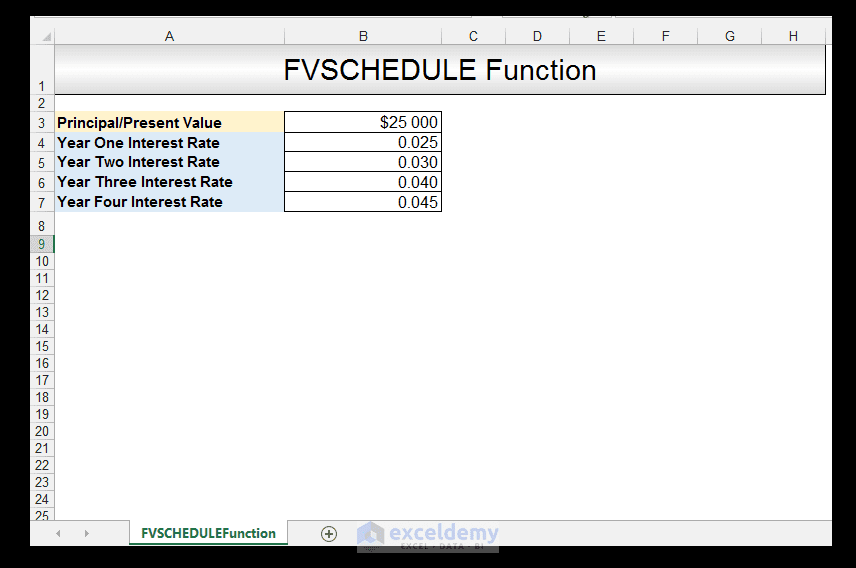2) So, in Cell B8, we input the following formula:

=FVSCHEDULE (B3, B4: B7)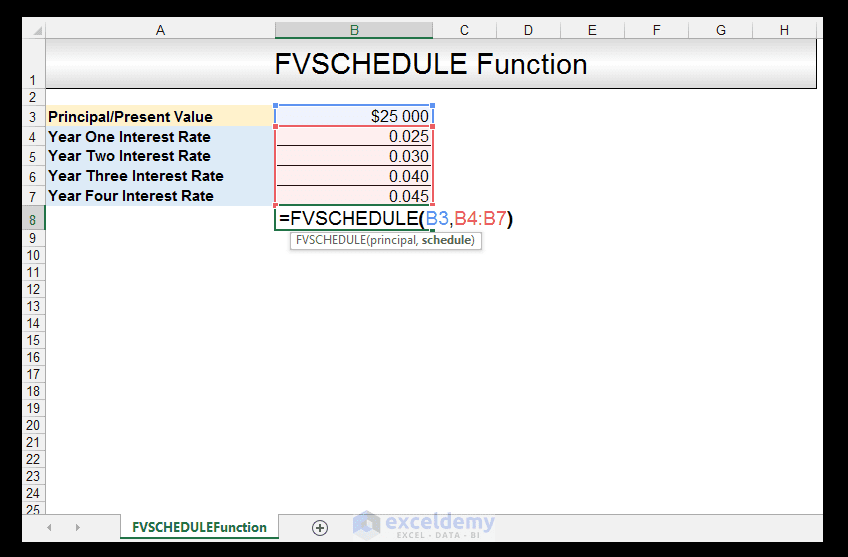3) Upon pressing CTRL-ENTER, we get the value of \$28 684.73, which means that the future value of an initial investment of \$25,000, over the four-year period, with interest rates of 2.5%, 3%, 4%, and 4.5% is \$28 684.73.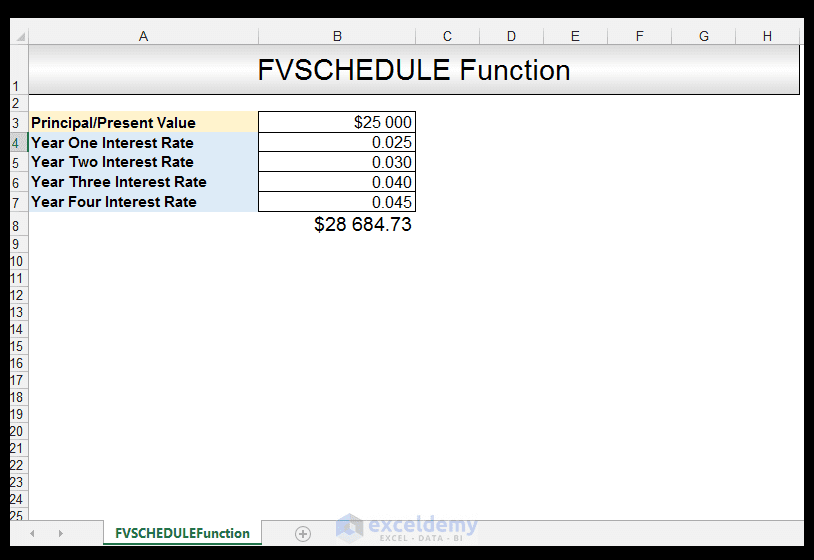4) Note that when one is hard coding the interest rates into the function, and not using cell references, you have to input the interest rates as an array of values. So, in Cell B10, enter the following formula making sure you surround the interest rates with curly braces:

=FVSCHEDULE (B3, {0.025, 0.030, 0.040, 0.045})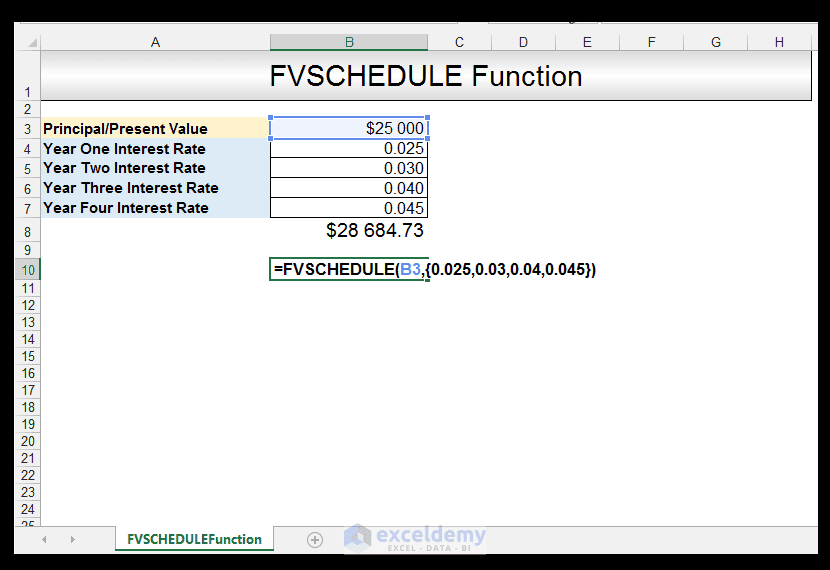Pressing either ENTER or CTRL-SHIFT-ENTER yields the same value of \$28 684.73.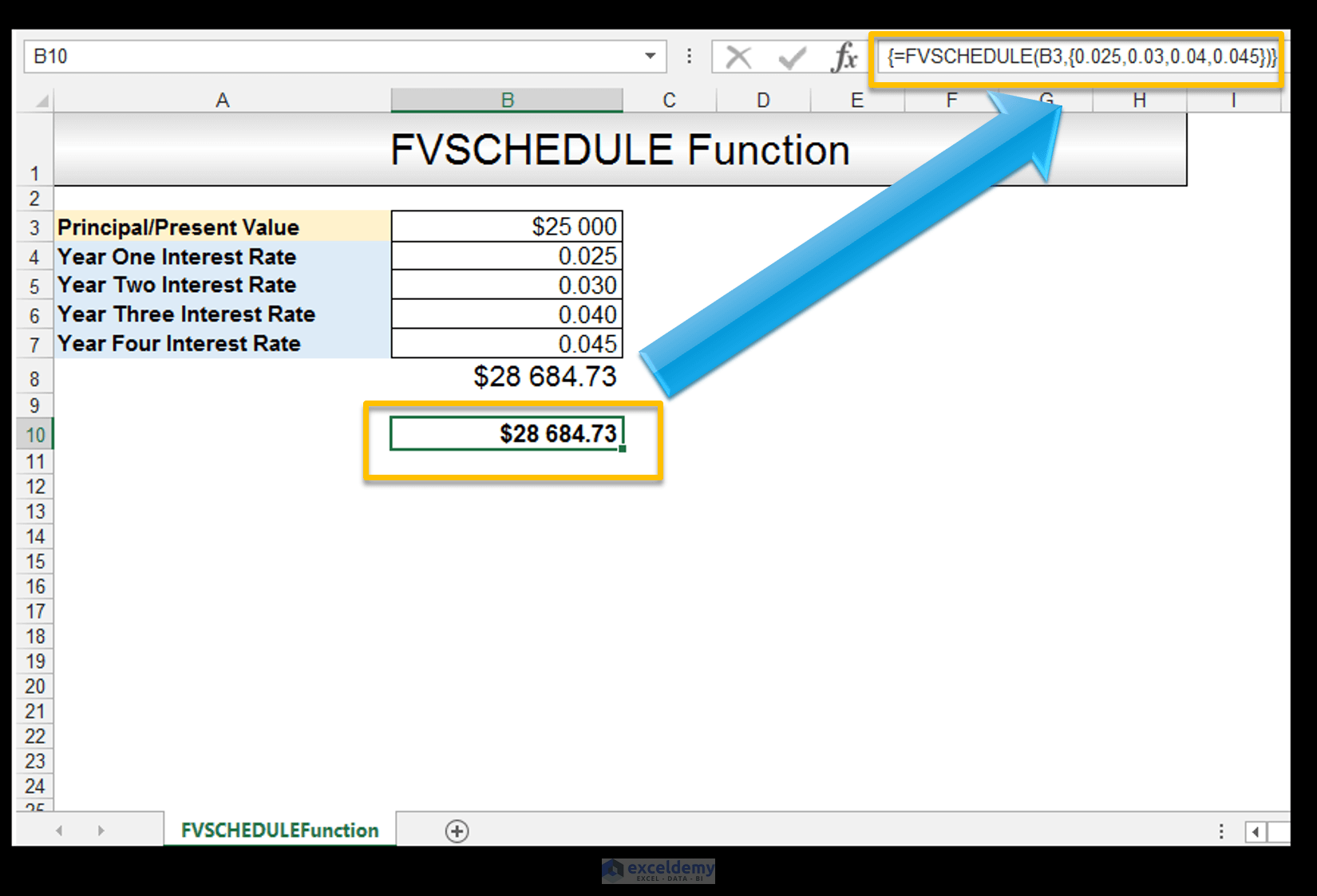And there you have it.

Conclusion

The FVSCHEDULE Function in Excel is a useful function that is utilized to calculate a future value, based on differing interest rates, which are not constant. If the interest rates, however, are constant and one has the information given for nper, pmt and/or pv and type, then one must utilize the FV Function in order to calculate the future value of an investment.

Please feel free to comment and tell us, if you use the FVSCHEDULE Function in your financial spreadsheets.

The PV, FV and PDURATION Excel Functions Tutorial

Calculating the Rate an Investment Achieves Using the RRI Function

How to Use the Excel MIRR Function

How to Use the DATEDIF Function in Excel